Anzeige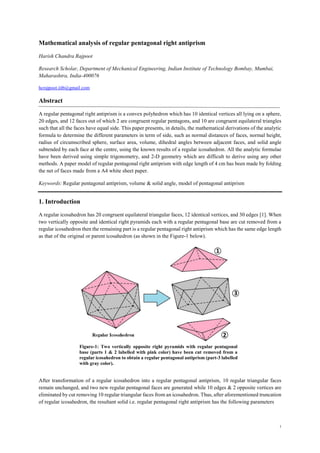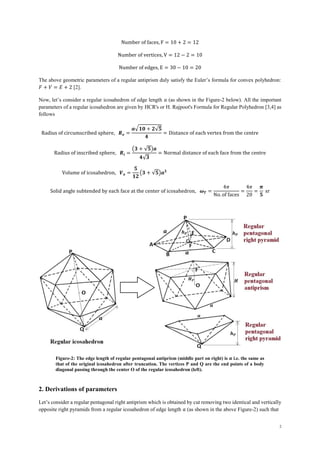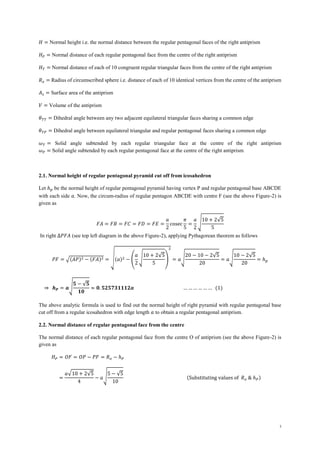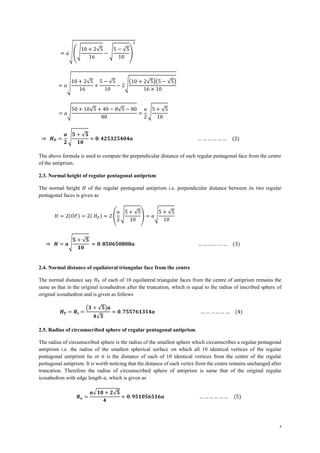Anzeige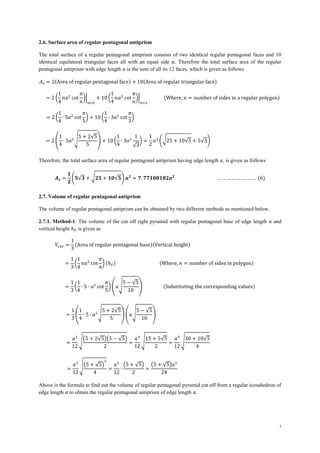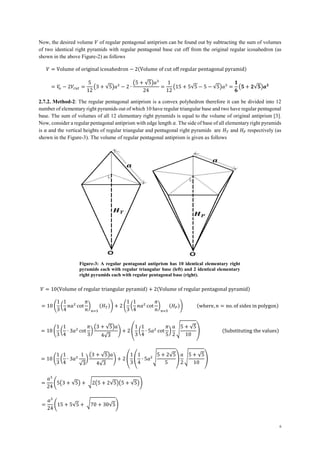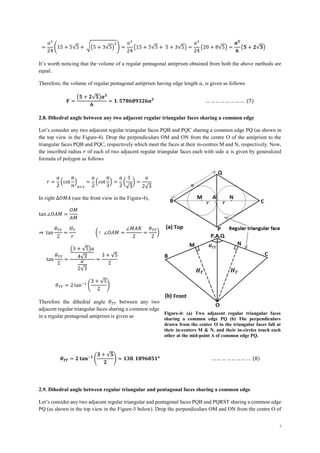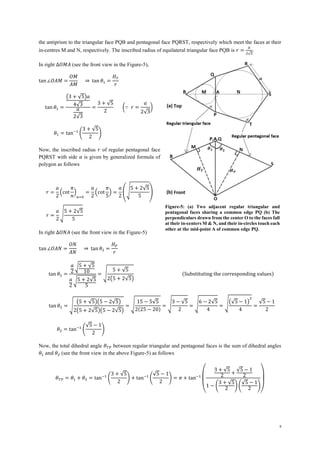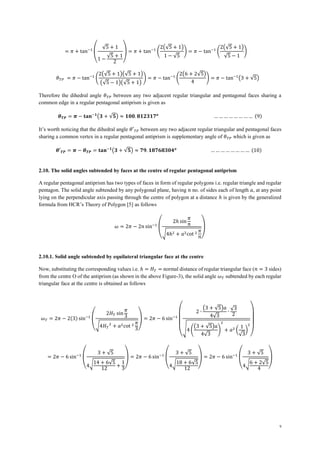Anzeige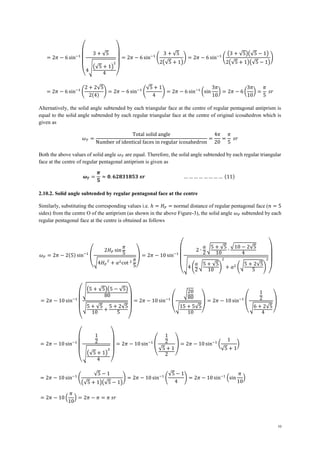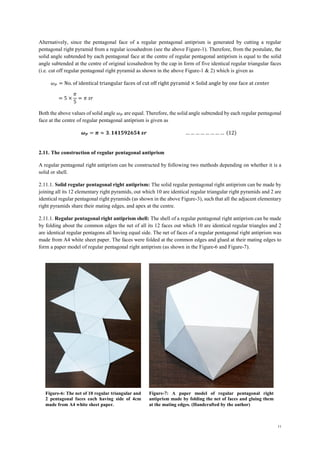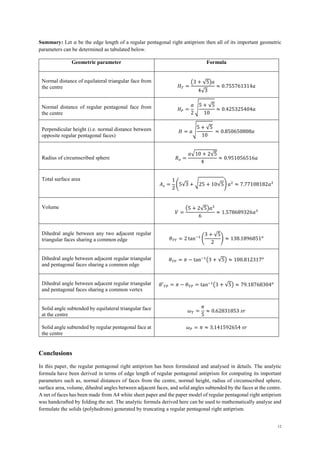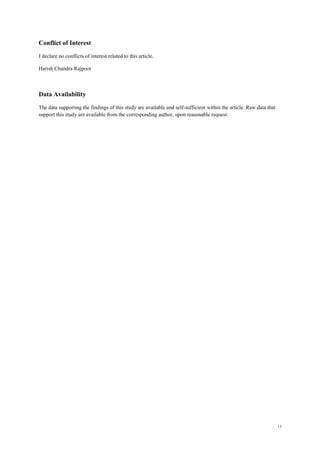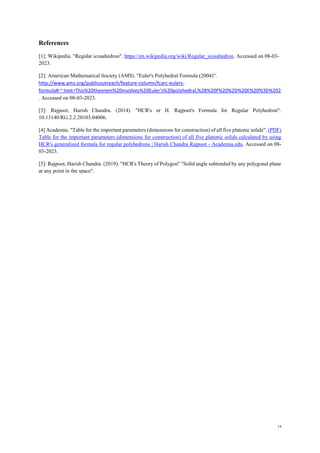Nächste SlideShare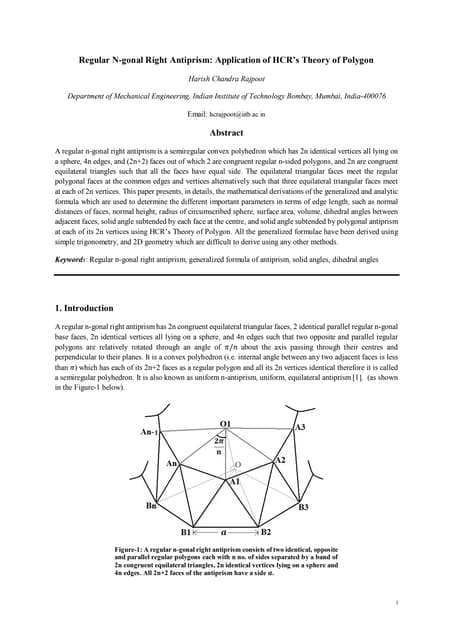Regular N-gonal Right Antiprism: Application of HCR’s Theory of Polygon
1 von 14
Anzeige

### Regular Pentagonal Right Antiprism by HCR

1. 1 Mathematical analysis of regular pentagonal right antiprism Harish Chandra Rajpoot Research Scholar, Department of Mechanical Engineering, Indian Institute of Technology Bombay, Mumbai, Maharashtra, India-400076 hcrajpoot.iitb@gmail.com Abstract A regular pentagonal right antiprism is a convex polyhedron which has 10 identical vertices all lying on a sphere, 20 edges, and 12 faces out of which 2 are congruent regular pentagons, and 10 are congruent equilateral triangles such that all the faces have equal side. This paper presents, in details, the mathematical derivations of the analytic formula to determine the different parameters in term of side, such as normal distances of faces, normal height, radius of circumscribed sphere, surface area, volume, dihedral angles between adjacent faces, and solid angle subtended by each face at the centre, using the known results of a regular icosahedron. All the analytic formulae have been derived using simple trigonometry, and 2-D geometry which are difficult to derive using any other methods. A paper model of regular pentagonal right antiprism with edge length of 4 cm has been made by folding the net of faces made from a A4 white sheet paper. Keywords: Regular pentagonal antiprism, volume & solid angle, model of pentagonal antiprism 1. Introduction A regular icosahedron has 20 congruent equilateral triangular faces, 12 identical vertices, and 30 edges . When two vertically opposite and identical right pyramids each with a regular pentagonal base are cut removed from a regular icosahedron then the remaining part is a regular pentagonal right antiprism which has the same edge length as that of the original or parent icosahedron (as shown in the Figure-1 below). After transformation of a regular icosahedron into a regular pentagonal antiprism, 10 regular triangular faces remain unchanged, and two new regular pentagonal faces are generated while 10 edges & 2 opposite vertices are eliminated by cut removing 10 regular triangular faces from an icosahedron. Thus, after aforementioned truncation of regular icosahedron, the resultant solid i.e. regular pentagonal right antiprism has the following parameters Figure-1: Two vertically opposite right pyramids with regular pentagonal base (parts 1 & 2 labelled with pink color) have been cut removed from a regular icosahedron to obtain a regular pentagonal antiprism (part-3 labelled with gray color).
2. 2 Number of faces, F = 10 + 2 = 12 Number of vertices, V = 12 − 2 = 10 Number of edges, E = 30 − 10 = 20 The above geometric parameters of a regular antiprism duly satisfy the Euler’s formula for convex polyhedron: 𝐹 + 𝑉 = 𝐸 + 2 . Now, let’s consider a regular icosahedron of edge length 𝑎 (as shown in the Figure-2 below). All the important parameters of a regular icosahedron are given by HCR's or H. Rajpoot's Formula for Regular Polyhedron [3,4] as follows Radius of circumscribed sphere, 𝑹𝒐 = 𝒂√𝟏𝟎 + 𝟐√𝟓 𝟒 = Distance of each vertex from the centre Radius of inscribed sphere, 𝑹𝒊 = (𝟑 + √𝟓)𝒂 𝟒√𝟑 = Normal distance of each face from the centre Volume of icosahedron, 𝑽𝒐 = 𝟓 𝟏𝟐 (𝟑 + √𝟓)𝒂𝟑 Solid angle subtended by each face at the center of icosahedron, 𝝎𝑻 = 4𝜋 No. of faces = 4𝜋 20 = 𝝅 𝟓 sr 2. Derivations of parameters Let’s consider a regular pentagonal right antiprism which is obtained by cut removing two identical and vertically opposite right pyramids from a regular icosahedron of edge length 𝑎 (as shown in the above Figure-2) such that Figure-2: The edge length of regular pentagonal antiprism (middle part on right) is 𝒂 i.e. the same as that of the original icosahedron after truncation. The vertices P and Q are the end points of a body diagonal passing through the center O of the regular icosahedron (left).
3. 3 𝐻 = Normal height i.e. the normal distance between the regular pentagonal faces of the right antiprism 𝐻𝑃 = Normal distance of each regular pentagonal face from the centre of the right antiprism 𝐻𝑇 = Normal distance of each of 10 congruent regular triangular faces from the centre of the right antiprism 𝑅𝑜 = Radius of circumscribed sphere i.e. distance of each of 10 identical vertices from the centre of the antiprism 𝐴𝑠 = Surface area of the antiprism 𝑉 = Volume of the antiprism 𝜃𝑇𝑇 = Dihedral angle between any two adjacent equilateral triangular faces sharing a common edge 𝜃𝑇𝑃 = Dihedral angle between equilateral triangular and regular pentagonal faces sharing a common edge 𝜔𝑇 = Solid angle subtended by each regular triangular face at the centre of the right antiprism 𝜔𝑃 = Solid angle subtended by each regular pentagonal face at the centre of the right antiprism 2.1. Normal height of regular pentagonal pyramid cut off from icosahedron Let ℎ𝑝 be the normal height of regular pentagonal pyramid having vertex P and regular pentagonal base ABCDE with each side 𝑎. Now, the circum-radius of regular pentagon ABCDE with centre F (see the above Figure-2) is given as 𝐹𝐴 = 𝐹𝐵 = 𝐹𝐶 = 𝐹𝐷 = 𝐹𝐸 = 𝑎 2 cosec 𝜋 5 = 𝑎 2 √ 10 + 2√5 5 In right ∆𝑃𝐹𝐴 (see top left diagram in the above Figure-2), applying Pythagorean theorem as follows 𝑃𝐹 = √(𝐴𝑃)2 − (𝐹𝐴)2 = √(𝑎)2 − ( 𝑎 2 √ 10 + 2√5 5 ) 2 = 𝑎√ 20 − 10 − 2√5 20 = 𝑎√ 10 − 2√5 20 = ℎ𝑝 ⇒ 𝒉𝑷 = 𝒂√ 𝟓 − √𝟓 𝟏𝟎 ≈ 𝟎. 𝟓𝟐𝟓𝟕𝟑𝟏𝟏𝟏𝟐𝒂 … … … … … … (1) The above analytic formula is used to find out the normal height of right pyramid with regular pentagonal base cut off from a regular icosahedron with edge length 𝑎 to obtain a regular pentagonal antiprism. 2.2. Normal distance of regular pentagonal face from the centre The normal distance of each regular pentagonal face from the centre O of antiprism (see the above Figure-2) is given as 𝐻𝑃 = 𝑂𝐹 = 𝑂𝑃 − 𝑃𝐹 = 𝑅𝑜 − ℎ𝑃 = 𝑎√10 + 2√5 4 − 𝑎√ 5 − √5 10 (Substituting values of 𝑅𝑜 & ℎ𝑃)
4. 4 = 𝑎√(√ 10 + 2√5 16 − √ 5 − √5 10 ) 2 = 𝑎√10 + 2√5 16 + 5 − √5 10 − 2√ (10 + 2√5)(5 − √5) 16 × 10 = 𝑎√ 50 + 10√5 + 40 − 8√5 − 80 80 = 𝑎 2 √ 5 + √5 10 ⇒ 𝑯𝑷 = 𝒂 𝟐 √ 𝟓 + √𝟓 𝟏𝟎 ≈ 𝟎. 𝟒𝟐𝟓𝟑𝟐𝟓𝟒𝟎𝟒𝒂 … … … … … … (2) The above formula is used to compute the perpendicular distance of each regular pentagonal face from the centre of the antiprism. 2.3. Normal height of regular pentagonal antiprism The normal height 𝐻 of the regular pentagonal antiprism i.e. perpendicular distance between its two regular pentagonal faces is given as 𝐻 = 2(𝑂𝐹) = 2( 𝐻𝑃) = 2 ( 𝑎 2 √ 5 + √5 10 ) = 𝑎√ 5 + √5 10 ⇒ 𝑯 = 𝒂√ 𝟓 + √𝟓 𝟏𝟎 ≈ 𝟎. 𝟖𝟓𝟎𝟔𝟓𝟎𝟖𝟎𝟖𝒂 … … … … … … (3) 2.4. Normal distance of equilateral triangular face from the centre The normal distance say 𝐻𝑇 of each of 10 equilateral triangular faces from the centre of antiprism remains the same as that in the original icosahedron after the truncation, which is equal to the radius of inscribed sphere of original icosahedron and is given as follows 𝑯𝑻 = 𝑹𝒊 = (𝟑 + √𝟓)𝒂 𝟒√𝟑 ≈ 𝟎. 𝟕𝟓𝟓𝟕𝟔𝟏𝟑𝟏𝟒𝒂 … … … … … … (4) 2.5. Radius of circumscribed sphere of regular pentagonal antiprism The radius of circumscribed sphere is the radius of the smallest sphere which circumscribes a regular pentagonal antiprism i.e. the radius of the smallest spherical surface on which all 10 identical vertices of the regular pentagonal antiprism lie or it is the distance of each of 10 identical vertices from the centre of the regular pentagonal antiprism. It is worth noticing that the distance of each vertex from the centre remains unchanged after truncation. Therefore the radius of circumscribed sphere of antiprism is same that of the original regular icosahedron with edge length 𝑎, which is given as 𝑹𝒐 = 𝒂√𝟏𝟎 + 𝟐√𝟓 𝟒 ≈ 𝟎. 𝟗𝟓𝟏𝟎𝟓𝟔𝟓𝟏𝟔𝒂 … … … … … … (5)
5. 5 2.6. Surface area of regular pentagonal antiprism The total surface of a regular pentagonal antiprism consists of two identical regular pentagonal faces and 10 identical equilateral triangular faces all with an equal side 𝑎. Therefore the total surface area of the regular pentagonal antiprism with edge length 𝑎 is the sum of all its 12 faces, which is given as follows 𝐴𝑠 = 2(Area of regular pentagonal face) + 10(Area of regular triangular face) = 2 ( 1 4 𝑛𝑎2 cot 𝜋 𝑛 )| 𝑛=5 + 10 ( 1 4 𝑛𝑎2 cot 𝜋 𝑛 )| 𝑛=3 (Where, 𝑛 = number of sides in a regular polygon) = 2 ( 1 4 ∙ 5𝑎2 cot 𝜋 5 ) + 10 ( 1 4 ∙ 3𝑎2 cot 𝜋 3 ) = 2 ( 1 4 ∙ 5𝑎2√ 5 + 2√5 5 ) + 10 ( 1 4 ∙ 3𝑎2 1 √3 ) = 1 2 𝑎2 (√25 + 10√5 + 5√3) Therefore, the total surface area of regular pentagonal antiprism having edge length 𝑎, is given as follows 𝑨𝒔 = 𝟏 𝟐 (𝟓√𝟑 + √𝟐𝟓 + 𝟏𝟎√𝟓) 𝒂𝟐 ≈ 𝟕. 𝟕𝟕𝟏𝟎𝟖𝟏𝟖𝟐𝒂𝟐 … … … … … … … … (6) 2.7. Volume of regular pentagonal antiprism The volume of regular pentagonal antiprism can be obtained by two different methods as mentioned below. 2.7.1. Method-1: The volume of the cut off right pyramid with regular pentagonal base of edge length 𝑎 and vertical height ℎ𝑃 is given as 𝑉𝑐𝑢𝑡 = 1 3 (Area of regular pentagonal base)(Vertical height) = 1 3 ( 1 4 𝑛𝑎2 cot 𝜋 𝑛 ) (ℎ𝑃) (Where, 𝑛 = number of sides in polygon) = 1 3 ( 1 4 ∙ 5 ∙ 𝑎2 cot 𝜋 5 ) (𝑎√ 5 − √5 10 ) (Substituting the corresponding values) = 1 3 ( 1 4 ∙ 5 ∙ 𝑎2√ 5 + 2√5 5 ) (𝑎√ 5 − √5 10 ) = 𝑎3 12 √ (5 + 2√5)(5 − √5) 2 = 𝑎3 12 √ 15 + 5√5 2 = 𝑎3 12 √ 30 + 10√5 4 = 𝑎3 12 √(5 + √5) 2 4 = 𝑎3 12 ∙ (5 + √5) 2 = (5 + √5)𝑎3 24 Above is the formula to find out the volume of regular pentagonal pyramid cut off from a regular icosahedron of edge length 𝑎 to obtain the regular pentagonal antiprism of edge length 𝑎.
6. 6 Now, the desired volume 𝑉 of regular pentagonal antiprism can be found out by subtracting the sum of volumes of two identical right pyramids with regular pentagonal base cut off from the original regular icosahedron (as shown in the above Figure-2) as follows 𝑉 = Volume of original icosahedron − 2(Volume of cut off regular pentagonal pyramid) = 𝑉 𝑜 − 2𝑉𝑐𝑢𝑡 = 5 12 (3 + √5)𝑎3 − 2 ∙ (5 + √5)𝑎3 24 = 1 12 (15 + 5√5 − 5 − √5)𝑎3 = 𝟏 𝟔 (𝟓 + 𝟐√𝟓)𝒂𝟑 2.7.2. Method-2: The regular pentagonal antiprism is a convex polyhedron therefore it can be divided into 12 number of elementary right pyramids out of which 10 have regular triangular base and two have regular pentagonal base. The sum of volumes of all 12 elementary right pyramids is equal to the volume of original antiprism . Now, consider a regular pentagonal antiprism with edge length 𝑎. The side of base of all elementary right pyramids is 𝑎 and the vertical heights of regular triangular and pentagonal right pyramids are 𝐻𝑇 and 𝐻𝑃 respectively (as shown in the Figure-3). The volume of regular pentagonal antiprism is given as follows 𝑉 = 10(Volume of regular triangular pyramid) + 2(Volume of regular pentagonal pyramid) = 10 ( 1 3 ( 1 4 𝑛𝑎2 cot 𝜋 𝑛 ) 𝑛=3 (𝐻𝑇)) + 2 ( 1 3 ( 1 4 𝑛𝑎2 cot 𝜋 𝑛 ) 𝑛=5 (𝐻𝑃)) (where, 𝑛 = no. of sides in polygon) = 10 ( 1 3 ( 1 4 ∙ 3𝑎2 cot 𝜋 3 ) (3 + √5)𝑎 4√3 ) + 2 ( 1 3 ( 1 4 ∙ 5𝑎2 cot 𝜋 5 ) 𝑎 2 √ 5 + √5 10 ) (Substituting the values) = 10 ( 1 3 ( 1 4 ∙ 3𝑎2 1 √3 ) (3 + √5)𝑎 4√3 ) + 2 ( 1 3 ( 1 4 ∙ 5𝑎2√ 5 + 2√5 5 ) 𝑎 2 √ 5 + √5 10 ) = 𝑎3 24 (5(3 + √5) + √2(5 + 2√5)(5 + √5)) = 𝑎3 24 (15 + 5√5 + √70 + 30√5) Figure-3: A regular pentagonal antiprism has 10 identical elementary right pyramids each with regular triangular base (left) and 2 identical elementary right pyramids each with regular pentagonal base (right).
7. 7 = 𝑎3 24 (15 + 5√5 + √(5 + 3√5) 2 ) = 𝑎3 24 (15 + 5√5 + 5 + 3√5) = 𝑎3 24 (20 + 8√5) = 𝒂𝟑 𝟔 (𝟓 + 𝟐√𝟓) It’s worth noticing that the volume of a regular pentagonal antiprism obtained from both the above methods are equal. Therefore, the volume of regular pentagonal antiprism having edge length 𝑎, is given as follows 𝑽 = (𝟓 + 𝟐√𝟓)𝒂𝟑 𝟔 ≈ 𝟏. 𝟓𝟕𝟖𝟔𝟖𝟗𝟑𝟐𝟔𝒂𝟑 … … … … … … … … (7) 2.8. Dihedral angle between any two adjacent regular triangular faces sharing a common edge Let’s consider any two adjacent regular triangular faces PQB and PQC sharing a common edge PQ (as shown in the top view in the Figure-4). Drop the perpendiculars OM and ON from the centre O of the antiprism to the triangular faces PQB and PQC, respectively which meet the faces at their in-centres M and N, respectively. Now, the inscribed radius 𝑟 of each of two adjacent regular triangular faces each with side 𝑎 is given by generalized formula of polygon as follows 𝑟 = 𝑎 2 (cot 𝜋 𝑛 ) 𝑛=3 = 𝑎 2 (cot 𝜋 3 ) = 𝑎 2 ( 1 √3 ) = 𝑎 2√3 In right ∆𝑂𝑀𝐴 (see the front view in the Figure-4), tan ∠𝑂𝐴𝑀 = 𝑂𝑀 𝐴𝑀 ⇒ tan 𝜃𝑇𝑇 2 = 𝐻𝑇 𝑟 (∵ ∠𝑂𝐴𝑀 = ∠𝑀𝐴𝑁 2 = 𝜃𝑇𝑇 2 ) tan 𝜃𝑇𝑇 2 = (3 + √5)𝑎 4√3 𝑎 2√3 = 3 + √5 2 𝜃𝑇𝑇 = 2 tan−1 ( 3 + √5 2 ) Therefore the dihedral angle 𝜃𝑇𝑇 between any two adjacent regular triangular faces sharing a common edge in a regular pentagonal antiprism is given as 𝜽𝑻𝑻 = 𝟐 𝐭𝐚𝐧−𝟏 ( 𝟑 + √𝟓 𝟐 ) ≈ 𝟏𝟑𝟖. 𝟏𝟖𝟗𝟔𝟖𝟓𝟏𝒐 … … … … … … … … (8) 2.9. Dihedral angle between regular triangular and pentagonal faces sharing a common edge Let’s consider any two adjacent regular triangular and pentagonal faces PQB and PQRST sharing a common edge PQ (as shown in the top view in the Figure-5 below). Drop the perpendiculars OM and ON from the centre O of Figure-4: (a) Two adjacent regular triangular faces sharing a common edge PQ (b) The perpendiculars drawn from the center O to the triangular faces fall at their in-centers M & N, and their in-circles touch each other at the mid-point A of common edge PQ.
8. 8 the antiprism to the triangular face PQB and pentagonal face PQRST, respectively which meet the faces at their in-centres M and N, respectively. The inscribed radius of equilateral triangular face PQB is 𝑟 = 𝑎 2√3 . In right ∆𝑂𝑀𝐴 (see the front view in the Figure-5), tan ∠𝑂𝐴𝑀 = 𝑂𝑀 𝐴𝑀 ⇒ tan 𝜃1 = 𝐻𝑇 𝑟 tan 𝜃1 = (3 + √5)𝑎 4√3 𝑎 2√3 = 3 + √5 2 (∵ 𝑟 = 𝑎 2√3 ) 𝜃1 = tan−1 ( 3 + √5 2 ) Now, the inscribed radius 𝑟 of regular pentagonal face PQRST with side 𝑎 is given by generalized formula of polygon as follows 𝑟 = 𝑎 2 (cot 𝜋 𝑛 ) 𝑛=5 = 𝑎 2 (cot 𝜋 5 ) = 𝑎 2 (√ 5 + 2√5 5 ) 𝑟 = 𝑎 2 √ 5 + 2√5 5 In right ∆𝑂𝑁𝐴 (see the front view in the Figure-5) tan ∠𝑂𝐴𝑁 = 𝑂𝑁 𝐴𝑁 ⇒ tan 𝜃2 = 𝐻𝑃 𝑟 tan 𝜃2 = 𝑎 2 √5 + √5 10 𝑎 2 √5 + 2√5 5 = √ 5 + √5 2(5 + 2√5) (Substituting the corresponding values) tan 𝜃2 = √ (5 + √5)(5 − 2√5) 2(5 + 2√5)(5 − 2√5) = √ 15 − 5√5 2(25 − 20) = √ 3 − √5 2 = √ 6 − 2√5 4 = √(√5 − 1) 2 4 = √5 − 1 2 𝜃2 = tan−1 ( √5 − 1 2 ) Now, the total dihedral angle 𝜃𝑇𝑃 between regular triangular and pentagonal faces is the sum of dihedral angles 𝜃1 and 𝜃2 (see the front view in the above Figure-5) as follows 𝜃𝑇𝑃 = 𝜃1 + 𝜃2 = tan−1 ( 3 + √5 2 ) + tan−1 ( √5 − 1 2 ) = 𝜋 + tan−1 ( 3 + √5 2 + √5 − 1 2 1 − ( 3 + √5 2 ) ( √5 − 1 2 ) ) Figure-5: (a) Two adjacent regular triangular and pentagonal faces sharing a common edge PQ (b) The perpendiculars drawn from the center O to the faces fall at their in-centers M & N, and their in-circles touch each other at the mid-point A of common edge PQ.
9. 9 = 𝜋 + tan−1 ( √5 + 1 1 − √5 + 1 2 ) = 𝜋 + tan−1 ( 2(√5 + 1) 1 − √5 ) = 𝜋 − tan−1 ( 2(√5 + 1) √5 − 1 ) 𝜃𝑇𝑃 = 𝜋 − tan−1 ( 2(√5 + 1)(√5 + 1) (√5 − 1)(√5 + 1) ) = 𝜋 − tan−1 ( 2(6 + 2√5) 4 ) = 𝜋 − tan−1 (3 + √5) Therefore the dihedral angle 𝜃𝑇𝑃 between any two adjacent regular triangular and pentagonal faces sharing a common edge in a regular pentagonal antiprism is given as 𝜽𝑻𝑷 = 𝝅 − 𝐭𝐚𝐧−𝟏 (𝟑 + √𝟓) ≈ 𝟏𝟎𝟎. 𝟖𝟏𝟐𝟑𝟏𝟕𝒐 … … … … … … … … (9) It’s worth noticing that the dihedral angle 𝜃′𝑇𝑃 between any two adjacent regular triangular and pentagonal faces sharing a common vertex in a regular pentagonal antiprism is supplementary angle of 𝜃𝑇𝑃 which is given as 𝜽′𝑻𝑷 = 𝝅 − 𝜽𝑻𝑷 = 𝐭𝐚𝐧−𝟏 (𝟑 + √𝟓) ≈ 𝟕𝟗. 𝟏𝟖𝟕𝟔𝟖𝟑𝟎𝟒𝒐 … … … … … … … … (10) 2.10. The solid angles subtended by faces at the centre of regular pentagonal antiprism A regular pentagonal antiprism has two types of faces in form of regular polygons i.e. regular triangle and regular pentagon. The solid angle subtended by any polygonal plane, having 𝑛 no. of sides each of length 𝑎, at any point lying on the perpendicular axis passing through the centre of polygon at a distance ℎ is given by the generalized formula from HCR’s Theory of Polygon  as follows 𝜔 = 2𝜋 − 2𝑛 sin−1 ( 2ℎ sin 𝜋 𝑛 √4ℎ2 + 𝑎2cot 2 𝜋 𝑛) 2.10.1. Solid angle subtended by equilateral triangular face at the centre Now, substituting the corresponding values i.e. ℎ = 𝐻𝑇 = normal distance of regular triangular face (𝑛 = 3 sides) from the centre O of the antiprism (as shown in the above Figure-3), the solid angle 𝜔𝑇 subtended by each regular triangular face at the centre is obtained as follows 𝜔𝑇 = 2𝜋 − 2(3) sin−1 ( 2𝐻𝑇 sin 𝜋 3 √4𝐻𝑇 2 + 𝑎2cot 2 𝜋 3) = 2𝜋 − 6 sin−1 ( 2 ∙ (3 + √5)𝑎 4√3 ∙ √3 2 √4 ( (3 + √5)𝑎 4√3 ) 2 + 𝑎2 ( 1 √3 ) 2 ) = 2𝜋 − 6 sin−1 ( 3 + √5 4√14 + 6√5 12 + 1 3) = 2𝜋 − 6 sin−1 ( 3 + √5 4√18 + 6√5 12 ) = 2𝜋 − 6 sin−1 ( 3 + √5 4√6 + 2√5 4 )
10. 10 = 2𝜋 − 6 sin−1 ( 3 + √5 4√(√5 + 1) 2 4 ) = 2𝜋 − 6 sin−1 ( 3 + √5 2(√5 + 1) ) = 2𝜋 − 6 sin−1 ( (3 + √5)(√5 − 1) 2(√5 + 1)(√5 − 1) ) = 2𝜋 − 6 sin−1 ( 2 + 2√5 2(4) ) = 2𝜋 − 6 sin−1 ( √5 + 1 4 ) = 2𝜋 − 6 sin−1 (sin 3𝜋 10 ) = 2𝜋 − 6 ( 3𝜋 10 ) = 𝜋 5 𝑠𝑟 Alternatively, the solid angle subtended by each triangular face at the centre of regular pentagonal antiprism is equal to the solid angle subtended by each regular triangular face at the centre of original icosahedron which is given as 𝜔𝑇 = Total solid angle Number of identical faces in regular icosahedron = 4𝜋 20 = 𝜋 5 𝑠𝑟 Both the above values of solid angle 𝜔𝑇 are equal. Therefore, the solid angle subtended by each regular triangular face at the centre of regular pentagonal antiprism is given as 𝝎𝑻 = 𝝅 𝟓 ≈ 𝟎. 𝟔𝟐𝟖𝟑𝟏𝟖𝟓𝟑 𝒔𝒓 … … … … … … … … (11) 2.10.2. Solid angle subtended by regular pentagonal face at the centre Similarly, substituting the corresponding values i.e. ℎ = 𝐻𝑃 = normal distance of regular pentagonal face (𝑛 = 5 sides) from the centre O of the antiprism (as shown in the above Figure-3), the solid angle 𝜔𝑃 subtended by each regular pentagonal face at the centre is obtained as follows 𝜔𝑃 = 2𝜋 − 2(5) sin−1 ( 2𝐻𝑃 sin 𝜋 5 √4𝐻𝑃 2 + 𝑎2cot 2 𝜋 5) = 2𝜋 − 10 sin−1 ( 2 ∙ 𝑎 2 √5 + √5 10 ∙ √10 − 2√5 4 √4 ( 𝑎 2 √5 + √5 10 ) 2 + 𝑎2 (√5 + 2√5 5 ) 2 ) = 2𝜋 − 10 sin−1 ( √(5 + √5)(5 − √5) 80 √5 + √5 10 + 5 + 2√5 5 ) = 2𝜋 − 10 sin−1 ( √20 80 √15 + 5√5 10 ) = 2𝜋 − 10 sin−1 ( 1 2 √6 + 2√5 4 ) = 2𝜋 − 10 sin−1 ( 1 2 √(√5 + 1) 2 4 ) = 2𝜋 − 10 sin−1 ( 1 2 √5 + 1 2 ) = 2𝜋 − 10 sin−1 ( 1 √5 + 1 ) = 2𝜋 − 10 sin−1 ( √5 − 1 (√5 + 1)(√5 − 1) ) = 2𝜋 − 10 sin−1 ( √5 − 1 4 ) = 2𝜋 − 10 sin−1 (sin 𝜋 10 ) = 2𝜋 − 10 ( 𝜋 10 ) = 2𝜋 − 𝜋 = 𝜋 𝑠𝑟
11. 11 Alternatively, since the pentagonal face of a regular pentagonal antiprism is generated by cutting a regular pentagonal right pyramid from a regular icosahedron (see the above Figure-1). Therefore, from the postulate, the solid angle subtended by each pentagonal face at the centre of regular pentagonal antiprism is equal to the solid angle subtended at the centre of original icosahedron by the cap in form of five identical regular triangular faces (i.e. cut off regular pentagonal right pyramid as shown in the above Figure-1 & 2) which is given as 𝜔𝑃 = No. of identical triangular faces of cut off right pyramid × Solid angle by one face at center = 5 × 𝜋 5 = 𝜋 𝑠𝑟 Both the above values of solid angle 𝜔𝑃 are equal. Therefore, the solid angle subtended by each regular pentagonal face at the centre of regular pentagonal antiprism is given as 𝝎𝑷 = 𝝅 ≈ 𝟑. 𝟏𝟒𝟏𝟓𝟗𝟐𝟔𝟓𝟒 𝒔𝒓 … … … … … … … … (12) 2.11. The construction of regular pentagonal antiprism A regular pentagonal right antiprism can be constructed by following two methods depending on whether it is a solid or shell. 2.11.1. Solid regular pentagonal right antiprism: The solid regular pentagonal right antiprism can be made by joining all its 12 elementary right pyramids, out which 10 are identical regular triangular right pyramids and 2 are identical regular pentagonal right pyramids (as shown in the above Figure-3), such that all the adjacent elementary right pyramids share their mating edges, and apex at the centre. 2.11.1. Regular pentagonal right antiprism shell: The shell of a regular pentagonal right antiprism can be made by folding about the common edges the net of all its 12 faces out which 10 are identical regular triangles and 2 are identical regular pentagons all having equal side. The net of faces of a regular pentagonal right antiprism was made from A4 white sheet paper. The faces were folded at the common edges and glued at their mating edges to form a paper model of regular pentagonal right antiprism (as shown in the Figure-6 and Figure-7). Figure-6: The net of 10 regular triangular and 2 pentagonal faces each having side of 4cm made from A4 white sheet paper. Figure-7: A paper model of regular pentagonal right antiprism made by folding the net of faces and gluing them at the mating edges. (Handcrafted by the author)
12. 12 Summary: Let 𝑎 be the edge length of a regular pentagonal right antiprism then all of its important geometric parameters can be determined as tabulated below. Geometric parameter Formula Normal distance of equilateral triangular face from the centre 𝐻𝑇 = (3 + √5)𝑎 4√3 ≈ 0.755761314𝑎 Normal distance of regular pentagonal face from the centre 𝐻𝑃 = 𝑎 2 √ 5 + √5 10 ≈ 0.425325404𝑎 Perpendicular height (i.e. normal distance between opposite regular pentagonal faces) 𝐻 = 𝑎√ 5 + √5 10 ≈ 0.850650808𝑎 Radius of circumscribed sphere 𝑅𝑜 = 𝑎√10 + 2√5 4 ≈ 0.951056516𝑎 Total surface area 𝐴𝑠 = 1 2 (5√3 + √25 + 10√5) 𝑎2 ≈ 7.77108182𝑎2 Volume 𝑉 = (5 + 2√5)𝑎3 6 ≈ 1.578689326𝑎3 Dihedral angle between any two adjacent regular triangular faces sharing a common edge 𝜃𝑇𝑇 = 2 tan−1 ( 3 + √5 2 ) ≈ 138.1896851𝑜 Dihedral angle between adjacent regular triangular and pentagonal faces sharing a common edge 𝜃𝑇𝑃 = 𝜋 − tan−1 (3 + √5) ≈ 100.812317𝑜 Dihedral angle between adjacent regular triangular and pentagonal faces sharing a common vertex 𝜃′𝑇𝑃 = 𝜋 − 𝜃𝑇𝑃 = tan−1 (3 + √5) ≈ 79.18768304𝑜 Solid angle subtended by equilateral triangular face at the centre 𝜔𝑇 = 𝜋 5 ≈ 0.62831853 𝑠𝑟 Solid angle subtended by regular pentagonal face at the centre 𝜔𝑃 = 𝜋 ≈ 3.141592654 𝑠𝑟 Conclusions In this paper, the regular pentagonal right antiprism has been formulated and analysed in details. The analytic formula have been derived in terms of edge length of regular pentagonal antiprism for computing its important parameters such as, normal distances of faces from the centre, normal height, radius of circumscribed sphere, surface area, volume, dihedral angles between adjacent faces, and solid angles subtended by the faces at the centre. A net of faces has been made from A4 white sheet paper and the paper model of regular pentagonal right antiprism was handcrafted by folding the net. The analytic formula derived here can be used to mathematically analyse and formulate the solids (polyhedrons) generated by truncating a regular pentagonal right antiprism.
13. 13 Conflict of Interest I declare no conflicts of interest related to this article. Harish Chandra Rajpoot Data Availability The data supporting the findings of this study are available and self-sufficient within the article. Raw data that support this study are available from the corresponding author, upon reasonable request.
14. 14 References : Wikipedia. "Regular icosahedron". https://en.wikipedia.org/wiki/Regular_icosahedron. Accessed on 08-03- 2023. : American Mathematical Society (AMS). "Euler's Polyhedral Formula (2004)". http://www.ams.org/publicoutreach/feature-column/fcarc-eulers- formula#:~:text=This%20theorem%20involves%20Euler's%20polyhedral,%2B%20F%20%2D%20E%20%3D%202 . Accessed on 08-03-2023. : Rajpoot, Harish Chandra. (2014). "HCR's or H. Rajpoot's Formula for Regular Polyhedron". 10.13140/RG.2.2.20103.04006.  Academia. "Table for the important parameters (dimensions for construction) of all five platonic solids". (PDF) Table for the important parameters (dimensions for construction) of all five platonic solids calculated by using HCR's generalized formula for regular polyhedrons | Harish Chandra Rajpoot - Academia.edu. Accessed on 08- 03-2023. : Rajpoot, Harish Chandra. (2019). "HCR's Theory of Polygon" "Solid angle subtended by any polygonal plane at any point in the space".
Anzeige